# 2.4   Case Study: Percolation

We conclude our study of functions and modules by considering a case study of developing a program to solve an interesting scientific problem. as Monte Carlo simulation to study a natural model known as percolation.

## Percolation.

We model the system as an n-by-n grid of sites. Each site is either blocked or open; open sites are initially empty. A full site is an open site that can be connected to an open site in the top row via a chain of neighboring (left, right, up, down) open sites. If there is a full site in the bottom row, then we say that the system percolates.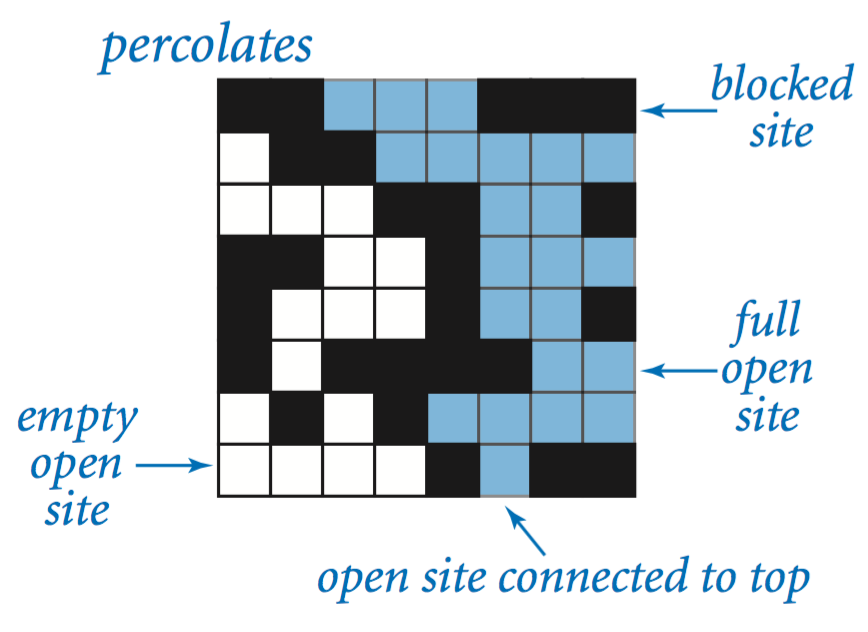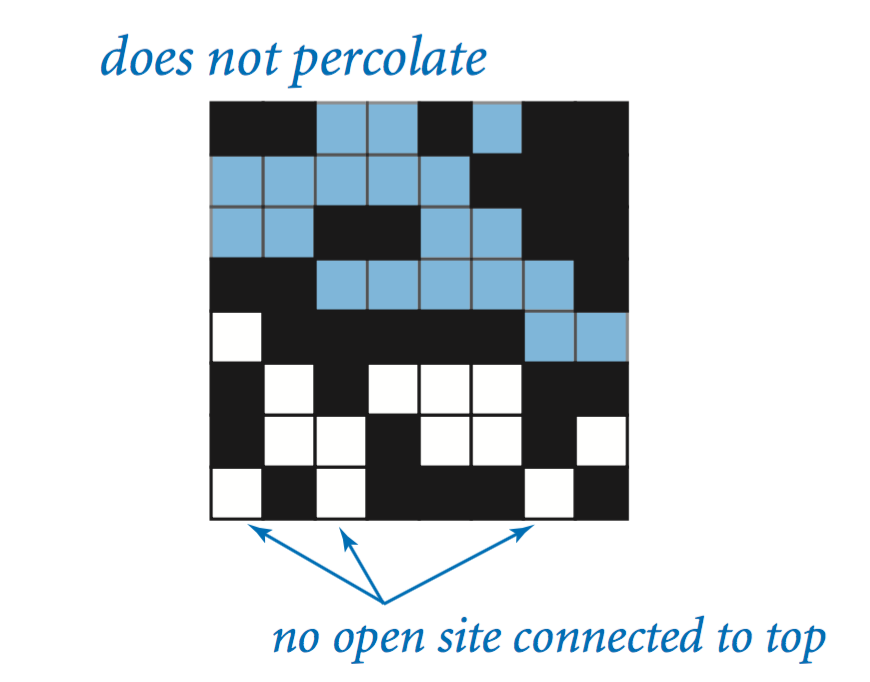If sites are independently set to be open with vacancy probability p, what is the probability that the system percolates? No mathematical solution to this problem has yet been derived. Our task is to write computer programs to help study the problem.

## Data representation.

Our first step is to pick a representation of the data. We use one boolean matrix isOpen[][] to represent which sites are open and another boolean matrix isFull[][] that to represent which sites are full.

## Vertical percolation.

Given a boolean matrix that represents the open sites, how do we figure out whether it represents a system that percolates? For the moment, we will consider a much simpler version of the problem that we call vertical percolation. The simplification is to restrict attention to vertical connection paths.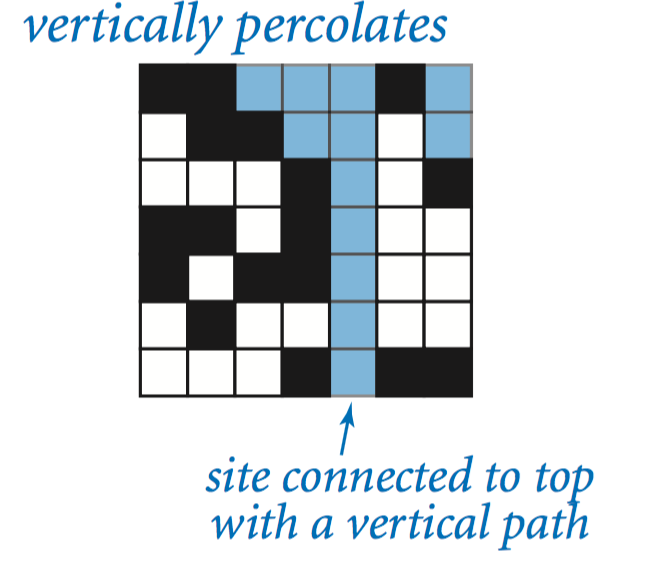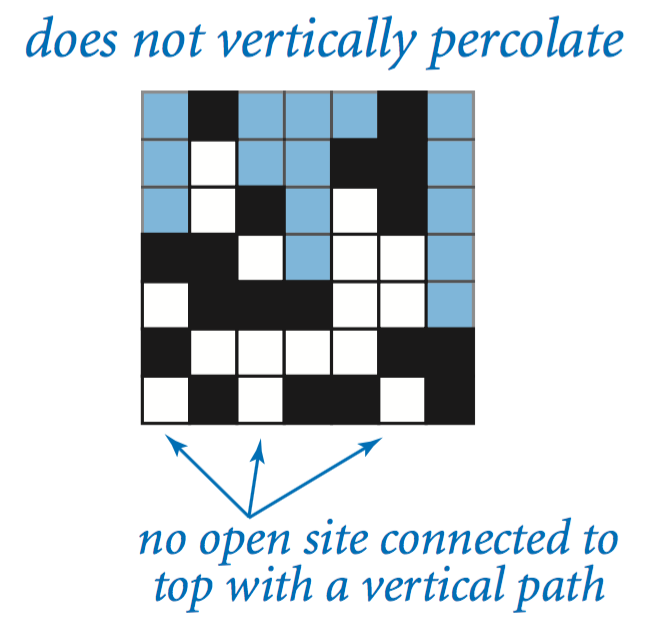VerticalPercolation.java determines the sites that are filled by some path that is connected vertically to the top using a simple calculation.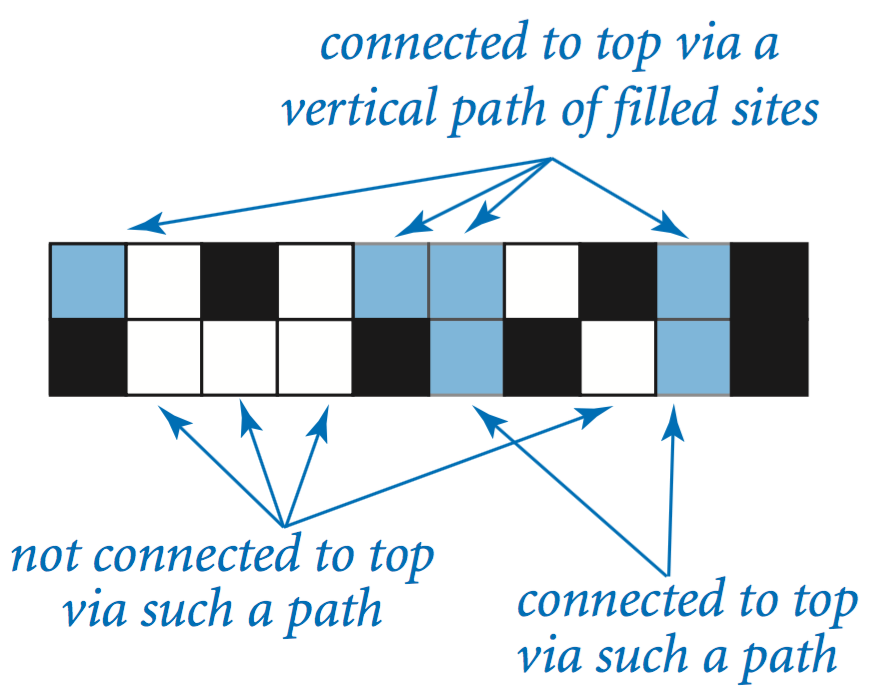## Data visualization.

PercolationVisualizer.java is a test client that generates random boolean matrices and plots them using standard drawing.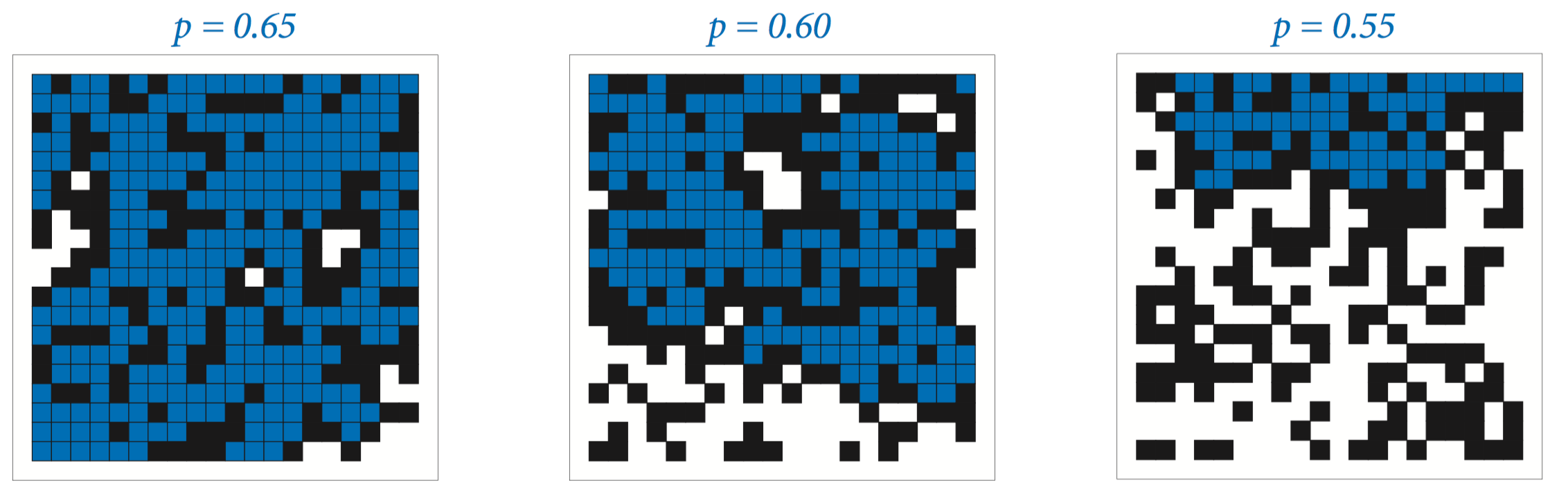## Estimating probabilities.

PercolationProbability.java estimates the probability that a random n-by-n system with site vacancy probability p percolates. We refer to this quantity as the percolation probability. To estimate its value, we simply run a number of experiments.

## Recursive solution for percolation.

How do we test whether a system percolates in the general case when any path starting at the top and ending at the bottom (not just a vertical one) will do the job? Remarkably, we can solve this problem with a compact program, based on a classic recursive scheme known as depth-first search. Percolation.java takes this approach. See the textbook for details.

PercolationPlot.java plots the percolation probability as a function of the site vacancy probability p for an n-by-n system. It uses a recursive approach that produces a good-looking curve at relatively low cost.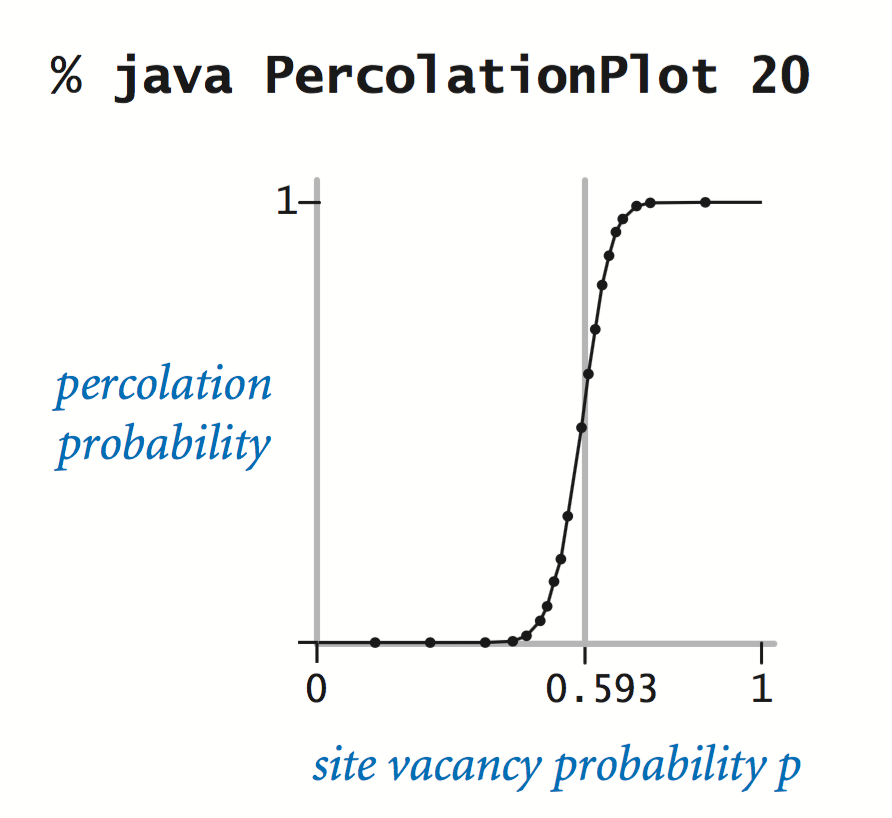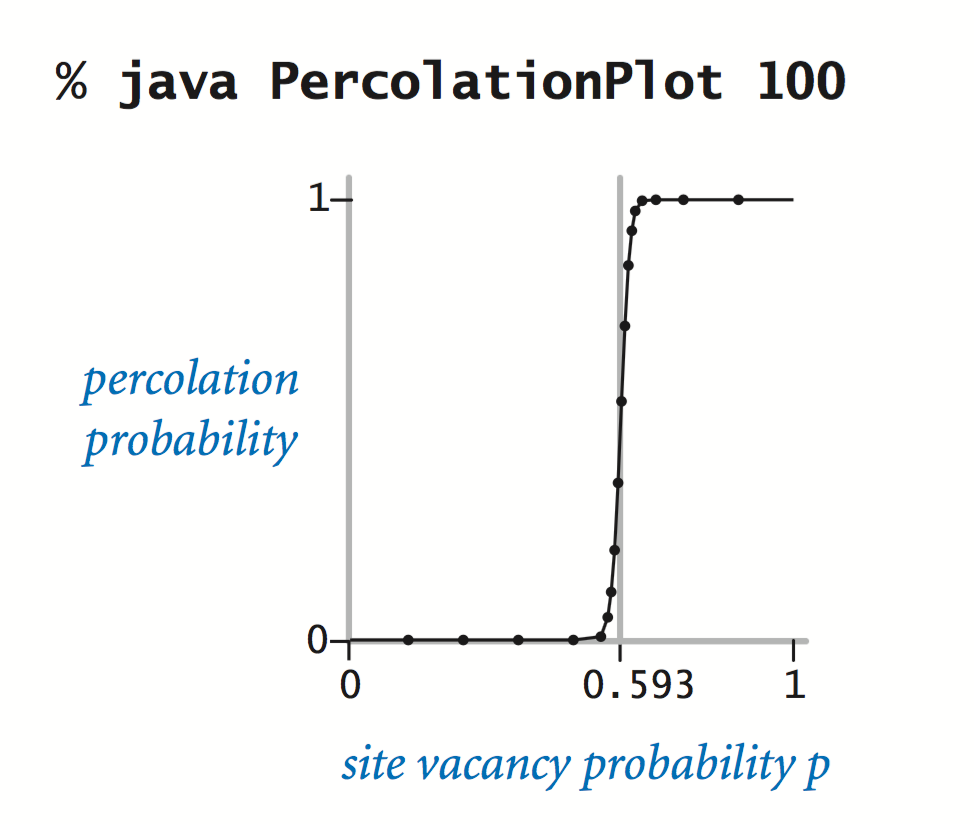The curves support the hypothesis that there is a threshold value (about 0.593): if p is greater than the threshold, then the system almost certainly percolates; if p is less than the threshold, then the system almost certainly does not percolate. As n increases, the curve approaches a step function that changes value from 0 to 1 at the threshold. This phenomenon, known as a phase transition, is found in many physical systems.

#### Exercises

1. Create a program PercolationDirected.java that tests for directed percolation (by leaving off the last recursive call in the recursive flow() method in Percolation.java, as described in the text), then use PercolationPlot.java to draw a plot of the directed percolation probability as a function of the site vacancy probability p.

#### Creative Exercises

1. Nonrecursive directed percolation. Write a nonrecursive program PercolationDirectedNonrecursive.java that tests for directed percolation by moving from top to bottom as in our vertical percolation code. Base your solution on the following computation: if any site in a contiguous subrow of open sites in the current row is connected to some full site on the previous row, then all of the sites in the subrow become full.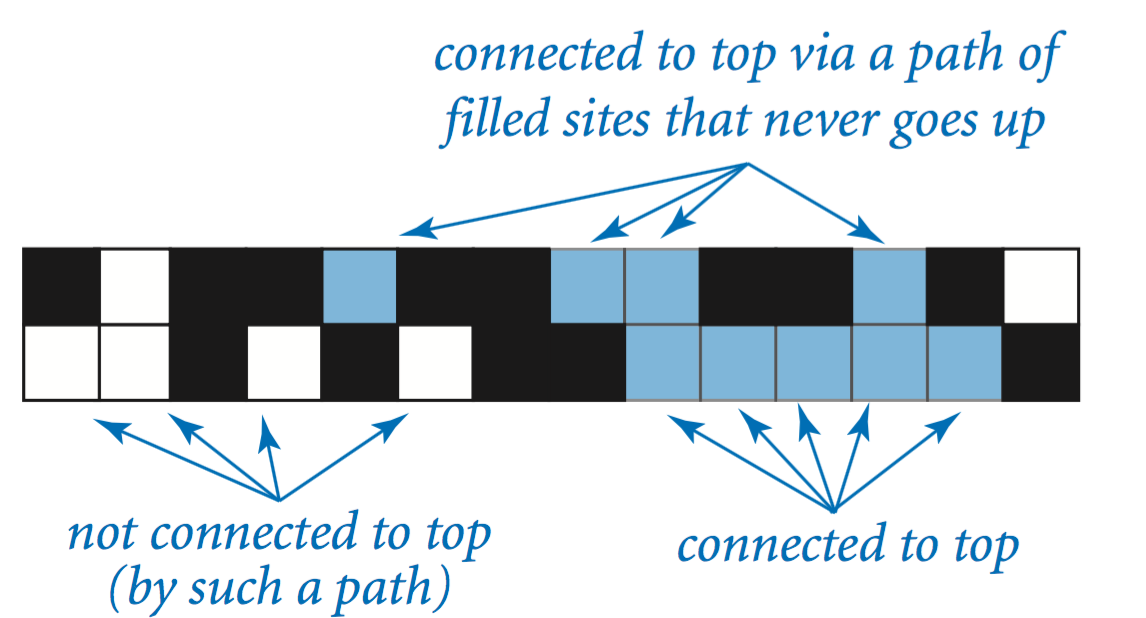#### Web Exercises

1. 2-by-2 percolation. Verify that the probability that a 2-by-2 system percolates is p^2 (2 - p^2), where p is the probability that a site is open.
2. Cubic curves. Use recursive subdivision algorithm to plot cubic curves.
3. Random walk. Term coined by Hungarian mathematician George Polya in a 1921 paper. Start at 0, go left with probability 1/2, go right with probability 1/2. Reflecting barrier at 0 - if particle hits 0, it must switch direction at next step and return to 1. Absorbing barrier at n - particle stops when it hits state n. Estimate number of steps as a function of n until the particle is absorbed.

Analytic solution: n2.

4. 3d random walk. Take a random walk on a 3d lattice, starting from (0, 0, 0). Write a program Polya.java to estimate the chance that you return to the origin after at most some number of steps, say 1,000. (In 1d and 2d, you will surely return; in 3d, there is a less than 50% chance.) Repeat the exercise on a 4d lattice. Solution: the actual probability (without the artificial restriction on the number of steps) is known as Polya's random walk constant. It is slightly more than 1/3 for 3d and slightly less than 1/5 for 4d.
5. Self-avoiding random walk. Simulate random walk on lattice until it intersects. Among all self-avoiding walks (SAW) of length n, what is average distance from origin? To save time in the simulation, exclude SAWs that ever take a step backwards. How long until at least one SAW of length n = 40, n = 80? What is half-life of a SAW? To save more time in the simulation, allow a SAW only to take a step into an unoccupied cell (and repeat until it gets trapped). Practically nothing is known rigorously about these questions, so simulation is the best recourse. Here's an article from the New Scientist.

Famous 1949 problem of Nobel prize winning chemist, Flory. Conjecture: exponent of root mean square displacement is 3/4 in 2D and 3/5 in 3D.

6. Self-avoiding random walk. Write a program SelfAvoidingWalk.java to simulate and animate a 2D self-avoiding random walk. Self-avoiding random walks arise in modeling physical processes like the folding of polymer molecules. Such walks are difficult to model using classical mathematics. so they are best studied by direct numerical simulation. See what fraction of such random walks end up more than R^2 (say 30) from the starting point.

Or keep a trail of length n.